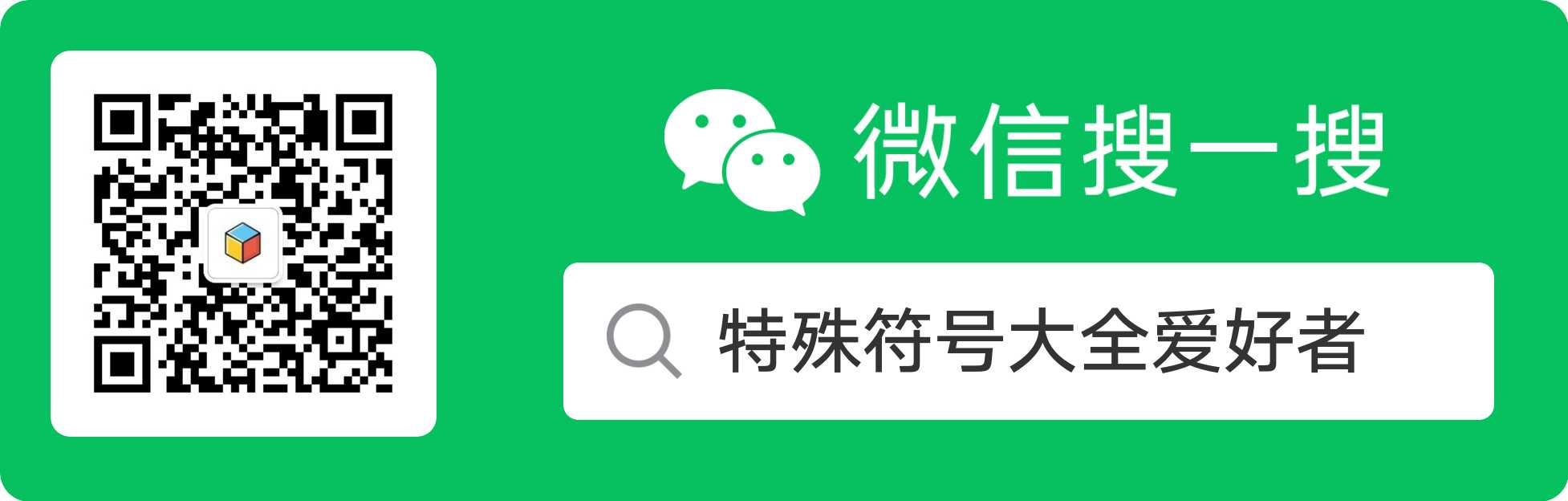# 表情符号大全

(•‾̑⌣‾̑•)✧˖° (๑´ڡ`๑) (๑¯ิε ¯ิ๑) (๑•́ ₃ •̀๑) ( ∙̆ .̯ ∙̆ ) (๑˘ ˘๑) (●′ω`●) ︶ε╰✿ ✿◡‿◡ (●･̆⍛･̆●)

=͟͟͞͞( •̀д•́) (눈_눈) (;´༎ຶД༎ຶ`) o‿≖✧ ಥ_ಥ

_(:qゝ∠)_ ((٩(//̀Д/́/)۶)) (´；ω；`) ( `)3')▃▃▃▅▆▇▉ v(ﾟｰﾟ) =≡Σ((( つ•̀ω•́)つ

(；′⌒`) ╰(*´︶`*)╯ ( ´´ิ∀´ิ` ) (´∩｀。) ( ื▿ ื) (｡ŏ_ŏ) ( •ิ _ •ิ ) ヽ(*΄◞ิ౪◟ิ‵ *)ﾉ ( ˘ ³˘)

(๑>m<๑) (; ´_ゝ`) (*ˉ﹃ˉ) (◍'౪`◍)ﾉﾞ (｡◝‿◜｡) (ಠ .̫.̫ ಠ) (´◞⊖◟`) (。≖ˇェˇ≖｡) ℰ⋆‿⋆ℰ

(ಠ .̫.̫ ಠ) p(´⌒｀｡q) ┗(´D`* (´D`*(´D`*)┛ <(▰˘◡˘▰)> (・ω< )★ (*ﾟｪﾟ*) ✧ (≖ ‿ ≖)✧

(◕ܫ◕) (｀◕‸◕´+) (▼ _ ▼) ( ◉ืൠ◉ื) ㄟ(◑‿◐ )ㄏ (●'◡'●)ﾉ♥ (｡◕ˇ∀ˇ◕） ~Ⴚ(●ტ●)Ⴢ~

⊙.⊙ ( ◔ ڼ ◔ ) ( ´◔ ‸◔`) (☍﹏⁰) (♥◠‿◠)ﾉ ✪ω✪ ʅ(‾◡◝)ʃ (,,Ծ‸Ծ,,) (๑°3°๑) ლ(╹◡╹ლ )

இwஇ (๑꒪◞౪◟꒪๑) (๑◕ܫ￩๑)b (*☉౪⊙*)　ヾ(o゜_,゜o)ノ ლↀѡↀლ (｡▰´◞◟｀▰｡)

( ͡° ͜ʖ ͡°) 　( ͡° ͜ʖ ͡°)✧ (*゜ロ゜)ノ 　 ( ´･∀･｀) °(°ˊДˋ°) ° (つ﹏⊂) --\(˙<>˙)/--

============================

┐( ¯¯ํ¯、¯¯ํ... •＜_• `<_` ˊ_ˋ =_.= 覀L覀 罒ω罒

┑(￣▽ ￣)┍ ┑(￣Д ￣)┍ ┑(￣。。￣)┍ ┐(—__—)┌ (≥﹏ ≤) (￣ε ￣)

╮(╯Д╰)╭ ╮(╯▽╰)╭ 乀(ˉεˉ乀) ～(￣▽￣～)(～￣▽￣)～ ヽ(ˋДˊ)ノ ヽ(ˋ▽ˊ)ノ

(*▔＾▔*) \(▔＾▔)/ ↖(▔＾▔)↗ ㄟ(▔,▔)ㄏ \(▔▽▔)/ ↖(▔▽▔)↗

ㄟ(▔▽▔)ㄏ ㄟ(▔＾▔ㄟ) (╯▔＾▔)╯ ㄟ(▔皿▔ㄟ) (╯▔皿▔)╯ ㄟ(▔（▔ㄟ)

(╯▔（▔)╯ ㄟ(▔▽▔ㄟ) (╯▔▽▔)╯ ㄟ(▔〔▔ㄟ) (╯▔〔▔)╯ (～o▔▽▔)～o o～(▔▽▔o～)

(╬▔＾▔)凸 (╬▔（▔)凸 (╬▔▽▔)凸 (╬▔皿▔)凸 (╬▔〔▔)凸

╮(〉_〉")╭ ╰(｀□′)╯ （＃－.－）/　 (）＾）)=凸 (((｀□′)) ╭(—?—)╮

ˋ(′～｀")ˊ ˋ(′o｀")ˊ ˋ(′ε｀")ˊ \(╯▼╰)/ <(｀^′)> ┌(｀▽′)╭ #(┬＿┬)

============================

⊙▂⊙　⊙0⊙　⊙＾⊙　⊙ω⊙　⊙﹏⊙　⊙△⊙　⊙▽⊙

?▂?　?0?　?＾?　?ω?　?﹏?　?△?　　?▽?

∩▂∩　∩0∩　∩＾∩　∩ω∩　∩﹏∩　∩△∩　∩▽∩

●▂●　　●0●　　●＾●　　●ω●　　●﹏●　　●△● 　●▽●

∪▂∪　∪0∪　∪＾∪　∪ω∪　∪﹏∪　∪△∪　∪▽∪

≥▂≤　≥0≤　≥＾≤　≥ω≤　≥﹏≤　≥△≤　≥▽≤

＞▂＜　＞0＜　＞＾＜　＞ω＜　＞﹏＜　＞△＜　＞▽＜

╯▂╰　╯0╰　╯＾╰　╯ω╰　╯﹏╰　╯△╰　╯▽╰

＋▂＋　＋0＋　＋＾＋　＋ω＋　＋﹏＋＋△＋　＋▽＋

ˋ▂ˊ　ˋ0ˊ　ˋ＾ˊ　ˋωˊ　ˋ﹏ˊ　ˋ△ˊ　ˋ▽ˊ

ˇ▂ˇ　ˇ0ˇ　ˇ＾ˇ　ˇωˇ　ˇ﹏ˇ　　ˇ△ˇ　ˇ▽ˇ

˙▂˙　˙0˙　˙＾˙　˙ω˙　˙﹏˙　˙△˙　˙▽˙

≡(▔﹏▔)≡ ⊙﹏⊙‖∣° ˋ＾ˊ〉-# ╯＾╰〉 (=｀′=)

<(｀^′)> 　o(?""?)o　(ˉ▽ˉ；)　(-__-)b　＼　＿　／

￣□￣｜｜　　------\\(˙<>˙)/------　　　<("""O""")>

(#｀′)凸 　(｀▽′)ψ 　（°ο°）~ @　　　(^人^)

(O ^ ~ ^ O) 　(＊?↓˙＊)　[>\/<]　↓。υ。↓

(*^〔^*) 　( /。\ ) 　　( ' – ' ) ( ^3^ )╱~~　 (；°○° ) ( > c < )

============================

<。)#)))≤ 烤鱼　(??)nnn 毛毛虫

\(0^◇^0)/ 麻雀

<*)>> >=< 鱼骨头　(=^ ^=) 猫　/(*w*)\ 兔子

≡[。。]≡ 螃蟹　(ˉ(∞)ˉ) 猪　■D" 咖啡杯

(:≡ 水母　(。。)～ 蝌蚪　ε==3 骨头

<□:≡ 乌贼　<｀▽′> 老虎　○●○— 烤丸子

(:◎)≡ 章鱼　ζ。≡ 狮子　(●-●) 太阳眼镜

@/" 蜗牛　∑^)/ 乌鸦　--<-<-<@ 玫瑰花

(((●< 蟑螂　(=^ω^=) 狐狸　＜※ 花束

============================

■D″咖啡杯 ∠※ 花束　　 8＜ 小剪刀

============================

<(￣）￣)> []~(￣▽￣)~* (￣﹏￣) (￣ˇ￣)

\(￣）￣)> <(￣）￣)/ (｀﹏′) ╮(｀▽′)╭

\(｀▽′)/

============================

≥▽≤y (/≥▽≤/) (≥▽≤)y (/≥▽≤)/~┴┴ (≥▽≤)(@)

\(≥3≤)/ \(≥ω≤)/ o(≥ω≤)o o(≥o≤)o ˋ( ° ▽、° )

o(≥v≤)o ┴┴~\(≥▽≤)/~┴┴ ≥□≤○

============================

《⊙＿⊙《 (⊙＿⊙) (@口@) ∑(@)(￣?￣)+ ∑(⊙▽⊙"a

(@[]@!!) ㄟ(≥◇≤)ㄏ(￣ε(#￣) ∑( ° △ °|||)︴

===========================

(￣▽￣)~* (￣▽￣)" ╮(╯▽╰)╭ ╮(￣▽￣)╭

=￣ω￣= (￣3￣)a ￣▽￣ ╮(╯3╰)╭

╮(╯3╰)╭ ╮(╯_╰)╭ (= 0 =)y o(）＾）)o

(￣.￣) \(￣< ￣)> (～o￣▽￣)～o ~。。。(~￣▽￣)~[]

============================

└(^o^)┘; 偶头好状壮ㄋㄟ```

﹌○﹋ 喔嗨呦^ˇ^≡

=^_^= 温馨的微笑!!!

Y(^_^)Y 举双手胜利

\^o^/ 欢呼

^o^y 胜利^o^y

↖(^ω^)↗ 小猪为你打气！

~^o^~ 加油呦!

*^?^* 笑，打

*^ο^* 哦～，呵呵傻笑

*^◎^* 呵呵大笑（嘴唇好厚）

*^÷^* 得意的笑（有上下唇的哟）

~~~^_^~~~ 笑毙罗（笑得连眼泪都蹦出来了...）

(-.-)=3 松ㄌ一口气~

~~~///(^v^)\\\~~~ 微笑表示友善!!!~哈~哈~

~@^_^@~ 可爱呦!

\\*^o^*//]\\*^o^*// 可爱ㄋㄟ~

~*.*~ 害羞又迷人的小女生

#^_^# 脸红了!!

∩__∩y 耶~~^^ (装可爱?!)

（*^＠^*） 乖～（还含个奶嘴哦） X﹏X糟糕．．完蛋的意思呀~~

(° ο°)~@ 晕倒了..

{{{(>_<)}}} 发抖

╯﹏╰ 粉无奈~~

\(╯-╰)/ 很没劲/无耐的意思

（╯＾╰〉 一脸苦瓜

｝_｝ 粉无奈..粉悲情

-____-" 唉~~别提了.....

。_。 受到打击，表情呈现呆滞样～

（*@＾@*〉 悲，晕

-（- 好伤心.

//(ㄒoㄒ)// 流泪中...

::>_<:: 哭

〒_〒 鸣~~我在哭...

%>_<% 我要哭了哦...

╰_╯我发火了!! 生气的意思~~~眉毛都翘起来~~

>_<# 粉生气~冒青筋

@x@ 生气

（ˋ＾ˊ〉-# 生气

（＞﹏＜） 不～

（*+﹏+*）~ @ 受不了～

x__x, 唉..... 别哭了!

>_<||| 很尴尬~!!!

^_^; 尴尬

⊙﹏⊙‖∣° 真尴尬～～

^_^||| 好尴尬！

^_^" 尴尬的笑.....

【→_→ (←_←)怀疑的眼神~~ 】

..@_@|||||.. 头昏眼花

…(⊙_⊙;)… ○圭~○列~~怎麼酱？

o_o .... 沉思(说穿了"呆滞状况")

O__O" 呆滞的眼神~~~!!

///^_^....... 造成"冷"...乾笑~

?o?||| 听无(就是听不懂ㄉ意思啦^^)

( ^_^ )? 什麼意思??

(+_+)? 不知道你在什麼

（?ε?）? 哩公啥哇听无啦~

o_O??? 发生啥事?

@_@a 搔头，疑惑

>"<|||| 伤脑筋的意思~ ‘(*>﹏<*)′ 好刺激..

（*@ο@*） 哇～

O_o 惊讶~~~

（⊙o⊙） 目瞪口呆

（°ο°）~ @ 晕倒，不省人事

*@_@* 崇拜的眼神;眼睛为之一亮

★~★ 见到偶像眼睛为之一亮

^◎- 爱你呦~~

(*^‧^*) 啵一个!

(^_^)∠※ 送你一束花

（*^〔^*〕 羞羞

‘（*^﹏^*）′ 超级羞羞

‘（*∩_∩*）′ 献上

~_~ 想睡觉了的意思!!

(～ o ～)~zZ 我想睡啦～

π_π 打瞌睡

=@~@= 一个看到美眉就紧张+脸红的大学生。

@(一-一) 秘密--------嘘```````

（ˇ＾ˇ〉 不以为然

(^人^) 拜托啦^^~

(^_^)/~~ 掰掰~

*x*... ... 你逼我也没用,我劫对不会搜粗弃的!

~w_w~ ... ... 嘘!别吵偶,让偶思考一下!

（ˇˍˇ） 嗯～，思考中

．．<{=．．． 乌鸦乌鸦飞过去表示冷场,无趣.....

（*>.<*）~ @ 酸～

~`o`~ 了解

ˋ_ˊ* 你不要命啦!!!

-.- 装蒜 ~>_<~+ 好感人唷...

Q_Q 流眼泪的样子!!

（＋﹏＋） 流泪，感动

‘（＋﹏＋）′ 超级感动，眼泪不停

^_^o ~~~ 感动感动~~~

============================

<(￣）￣)> <(￣）￣)><(￣）￣)>

<(￣）￣)/<(￣）￣)/<(￣）￣)/

o(╬￣皿￣)=○# (￣#)3￣) 看拳

K.O <(o一-一)=○# (￣#)3￣)

(╯｀□′)╯（ ┴—┴ 翻桌啦!

╭∩╮(￣▽￣)╭∩╮你有没有搞错!

\("▔□▔)/\("▔□▔)/\("▔□▔)/

~(￣▽￣)~(￣▽￣)~爽到不行~

~(￣3￣)~(〕ε〉)~(￣3￣)~快送医!

<( ￣^￣)（θ（θ☆( >_<) 无影脚

<( ｀□′)———Cε(┬＿┬)3 笨蛋

<( ｀□′)———C＜—___-)|| 夹!

╭ (′▽`)╭(′▽`)╭(′▽`)╯Go!

＾(￣）￣)《(￣）￣)＾飞.飞.飞.

<(￣﹌￣)@m 凶手!凶手就是你!

╭(﹊∩∩﹊#)╮ 我..我..是大猪头

╮(╯◇╰)╭口禾火～口禾火～ 来嘛!

…(⊙_⊙;)… ○圭~○列~怎麼酱？

<(￣oo,￣)/猪头不是一天造成的!

ˋ(′o｀")ˊ这个你问我也不知道~

\("▔□▔)/\("▔□▔)/ 有火星人~

┌(｀▽′)╭ 不要以为我不知道咩!

<(￣ c￣)y▂ξ 真烦,来哈根草吧~

（＃－.－）/ 叔叔~这样很冷耶!

\(￣▽￣)♂ 我是优质大帅哥一枚.

♀(￣▽￣)/ 我是优质大美女一枚.

┐(—__—)┌ 你说我有啥米办法咧~

○(*￣）￣*)○ 吃饱饱，睡好好!

○(#｀＾′#)○ 有没有被猪揍过啊?

ε(┬┬＿┬┬)3 我 真 命 苦 ..

┴┴ （╰(｀□′)╯（ ┴┴ 拆屋

≡￣﹏￣≡ 冷到不行..

<(｀^′)> 我看你还是回火星去好了!

<(￣oo,￣)/ 没看过猪哥吗??...

<(￣）￣)/ 喜欢吗？把拔买给你～

＾(￣）￣)＾ 这学期欧趴欧趴啦～

<( ￣^￣)（θ（θ（θ（θ（θ（θ（θ（θ（θ☆( >_<)~啊! 无影脚升级版

o(｀▽′)ψ 恶魔集团

ψ(╰_╯)σ??☆咒

ψ(￣）￣)ψ(￣）￣)ψ

(￣)＾(￣) 嘟著嘴

（⊙o⊙） 目瞪口呆

\(～__～)/ 要抱抱啦...

(＞﹏＜) 不～要～啦

（⊙.⊙）a...怎样？

〒▽〒 哇哇～人家不依

o(一＾一+)o 怨.念

(—.—|||| 很多条线

（＃－－）/ 下次小心.

ㄟ(川.一ㄟ) 鬼魂团

√(—皿—)√ 让我咬

(′ 3`)y==~ 人生海海..

(￣y▽￣)╭ 唉唷唷~

\(╯-╰)/ 不是我杀的

(￣▽￣＃) = ﹏﹏ 飘走

m(_ _)m大人饶命啊!

╭(′▽`)╭(′▽`)╯(让咱们一起奔向夕阳吧...)